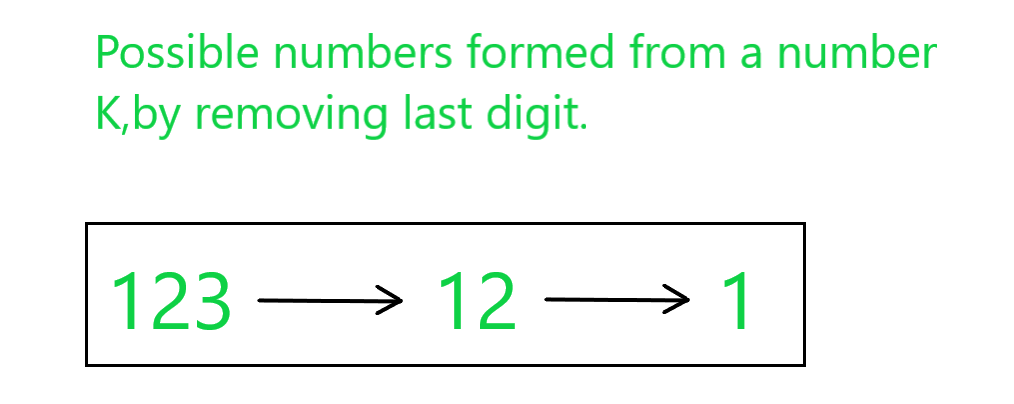Skip to content
Related Articles
Find a number K having sum of numbers obtained by repeated removal of last digit of K is N
• Difficulty Level : Hard
• Last Updated : 26 May, 2021

Given an integer N, the task is to find an integer K such that the sum of the numbers formed by repeated removal of the last digit of K is equal to N.

Examples:

Input: N = 136
Output: 123
Explanation:The numbers formed by repeatedly removing the last digit of 123 are {123, 12, 1}.
Therefore, the sum of these numbers = 123 + 12 + 1 = 136( = N).

Input: N = 107
Output: 98
Explanation:
The numbers formed by repeatedly removing the last digit of 98 are {98, 9}.
Therefore, the sum of these numbers = 98 + 7 = 107( = N).

Approach: The approach is based on the following observations:

• Consider K = 123.
• The possible numbers formed from 123 are 1, 12, and 123.
• Now, 123 can be expressed as 100 + 20 + 3. If all the other numbers are expressed similarly, then the idea is to know the position and frequency of each digit in all the numbers combined, to get the total sum as N

• Now, for the given number N of length L. Divide the number with L number of 1s to get the highest place digit.
• Calculate the remainder which will be our newly formed N.
• Again divide the newly formed N with (L – 1) number of 1s to get 2nd highest place digit and continue till the L becomes 0.

Follow the steps below to solve the problem:

• Let L be the count of digits in the given number N.
• Initialize string str as L numbers of 1s in it.
• Initialize a variable ans as zero that will store the resultant number K.
• Iterate until the string str is not empty and follow the steps below:

ans = ans*10 + (N/M)

• Update N to N % M.
• Remove the last character from the string str.
• After the above steps, print the value stored in the ans which is the required value of K.

Below is the implementation of the above approach:

## C++

 `// C++ program for the above approach` `#include ``using` `namespace` `std;` `// Function to find the value of K``int` `findK(``int` `N, ``int` `l)``{``    ``// Stores l number of 1s``    ``string ones = ``""``;` `    ``while` `(l--) {` `        ``// Storing 1's``        ``ones = ones + ``'1'``;``    ``}` `    ``// Stores the value of K``    ``int` `ans = 0;` `    ``// Iterate until ones is empty``    ``while` `(ones != ``""``) {` `        ``// Convert ones to number``        ``int` `m = stoi(ones);` `        ``// Update the ans``        ``ans = (ans * 10) + (N / m);` `        ``// Update N to N%m``        ``N = N % m;` `        ``// Removing last digit from ones``        ``ones.pop_back();``    ``}` `    ``// Return the value of K``    ``return` `ans;``}` `// Driver Code``int` `main()``{``    ``// Given number N``    ``int` `N = 136;` `    ``// Number of digits in N``    ``int` `L = to_string(N).length();` `    ``// Funtion Call``    ``cout << findK(N, L);` `    ``return` `0;``}`

## Java

 `// Java program for``// the above approach``import` `java.util.*;``class` `GFG{` `// Function to find the``// value of K``static` `int` `findK(``int` `N,``                 ``int` `l)``{``  ``// Stores l number of 1s``  ``String ones = ``""``;` `  ``while` `(l-- > ``0``)``  ``{``    ``// Storing 1's``    ``ones += ``'1'``;``  ``}` `  ``// Stores the value of K``  ``int` `ans = ``0``;` `  ``// Iterate until ones is empty``  ``while` `(!ones.equals(``""``))``  ``{``    ``// Convert ones to number``    ``int` `m = Integer.valueOf(ones);` `    ``// Update the ans``    ``ans = (ans * ``10``) + (N / m);` `    ``// Update N to N%m``    ``N = N % m;` `    ``// Removing last digit from ones``    ``ones = ones.substring(``0``,``           ``ones.length() - ``1``);``  ``}` `  ``// Return the value of K``  ``return` `ans;``}` `// Driver Code``public` `static` `void` `main(String[] args)``{``  ``// Given number N``  ``int` `N = ``136``;` `  ``// Number of digits in N``  ``int` `L = String.valueOf(N).length();` `  ``// Funtion Call``  ``System.out.print(findK(N, L));``}``}` `// This code is contributed by 29AjayKumar`

## Python3

 `# Python3 program for``# the above approach` `# Function to find``# the value of K``def` `findK(N, l):` `    ``# Stores l number of 1s``    ``ones ``=` `""` `    ``while` `(l):` `        ``#  Storing 1's``        ``ones ``=` `ones ``+` `'1'``        ``l ``-``=` `1``   ` `    ``# Stores the value of K``    ``ans ``=` `0``    ` `    ``# Iterate until ones``    ``# is empty``    ``while` `(ones !``=` `""):` `        ``# Convert ones to number``        ``m ``=` `int``(ones)` `        ``# Update the ans``        ``ans ``=` `(ans ``*` `10``) ``+` `(N ``/``/` `m)` `        ``# Update N to N%m``        ``N ``=` `N ``%` `m` `        ``# Removing last digit from ones``        ``ones ``=` `ones.replace(ones[``-``1``], "", ``1``)``    ` `    ``# Return the value of K``    ``return` `ans` `# Driver Code``if` `__name__ ``=``=` `"__main__"``:``  ` `    ``# Given number N``    ``N ``=` `136` `    ``# Number of digits in N``    ``L ``=` `len``(``str``(N))` `    ``# Funtion Call``    ``print` `(findK(N, L))` `# This code is contributed by Chitranayal`

## C#

 `// C# program for``// the above approach``using` `System;``class` `GFG{` `// Function to find the``// value of K``static` `int` `findK(``int` `N,``                 ``int` `l)``{``  ``// Stores l number of 1s``  ``String ones = ``""``;` `  ``while` `(l-- > 0)``  ``{``    ``// Storing 1's``    ``ones += ``'1'``;``  ``}` `  ``// Stores the value of K``  ``int` `ans = 0;` `  ``// Iterate until ones is empty``  ``while` `(!ones.Equals(``""``))``  ``{``    ``// Convert ones to number``    ``int` `m = Int32.Parse(ones);` `    ``// Update the ans``    ``ans = (ans * 10) + (N / m);` `    ``// Update N to N%m``    ``N = N % m;` `    ``// Removing last digit from ones``    ``ones = ones.Substring(0,``           ``ones.Length - 1);``  ``}` `  ``// Return the value of K``  ``return` `ans;``}` `// Driver Code``public` `static` `void` `Main(String[] args)``{``  ``// Given number N``  ``int` `N = 136;` `  ``// Number of digits in N``  ``int` `L = String.Join(``""``, N).Length;` `  ``// Funtion Call``  ``Console.Write(findK(N, L));``}``}` `// This code is contributed by Princi Singh`

## Javascript

 ``
Output:
`123`

Time Complexity: O(log10N)
Auxiliary Space: O(1)

Attention reader! Don’t stop learning now. Get hold of all the important DSA concepts with the DSA Self Paced Course at a student-friendly price and become industry ready.  To complete your preparation from learning a language to DS Algo and many more,  please refer Complete Interview Preparation Course.

In case you wish to attend live classes with industry experts, please refer Geeks Classes Live

My Personal Notes arrow_drop_up